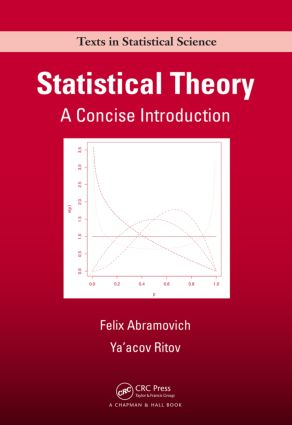# Statistical Theory

## A Concise Introduction, 1st Edition

Chapman and Hall/CRC

240 pages | 22 B/W Illus.

##### Purchasing Options:\$ = USD
Hardback: 9781439851845
pub: 2013-04-25
Currently out of stock
\$85.95
x

FREE Standard Shipping!

### Description

Designed for a one-semester advanced undergraduate or graduate course, Statistical Theory: A Concise Introduction clearly explains the underlying ideas and principles of major statistical concepts, including parameter estimation, confidence intervals, hypothesis testing, asymptotic analysis, Bayesian inference, and elements of decision theory. It introduces these topics on a clear intuitive level using illustrative examples in addition to the formal definitions, theorems, and proofs.

Based on the authors’ lecture notes, this student-oriented, self-contained book maintains a proper balance between the clarity and rigor of exposition. In a few cases, the authors present a "sketched" version of a proof, explaining its main ideas rather than giving detailed technical mathematical and probabilistic arguments. Chapters and sections marked by asterisks contain more advanced topics and may be omitted. A special chapter on linear models shows how the main theoretical concepts can be applied to the well-known and frequently used statistical tool of linear regression.

Requiring no heavy calculus, simple questions throughout the text help students check their understanding of the material. Each chapter also includes a set of exercises that range in level of difficulty.

### Reviews

"As teachers of theoretical statistics, we can use a new approach, which this text offers. … a helpful resource for teachers of mathematical statistics who are looking for an outline of teaching material and useable depth. Their material attains a workable syllabus, which can be easily augmented with the teacher’s preferred emphasis. This volume will make a solid contribution to any theoretical statistics instructor’s collection due to its convenient size, its scope of coverage, judicious use of examples, and clarity of exposition."

The American Statistician, May 2014

Introduction

Preamble

Likelihood

Sufficiency

Minimal sufficiency

Completeness

Exponential family of distributions

Point Estimation

Introduction

Maximum likelihood estimation

Method of moments

Method of least squares

Goodness-of-estimation. Mean squared error.

Unbiased estimation

Confidence Intervals, Bounds, and Regions

Introduction

Quoting the estimation error

Confidence intervals

Confidence bounds

Confidence regions

Hypothesis Testing

Introduction

Simple hypotheses

Composite hypotheses

Hypothesis testing and confidence intervals

Sequential testing

Asymptotic Analysis

Introduction

Convergence and consistency in MSE

Convergence and consistency in probability

Convergence in distribution

The central limit theorem

Asymptotically normal consistency

Asymptotic confidence intervals

Asymptotic normality of the MLE

Multiparameter case

Asymptotic distribution of the GLRT. Wilks’ theorem.

Bayesian Inference

Introduction

Choice of priors

Point estimation

Interval estimation. Credible sets.

Hypothesis testing

Elements of Statistical Decision Theory

Introduction and notations

Risk function and admissibility

Minimax risk and minimax rules

Bayes risk and Bayes rules

Posterior expected loss and Bayes actions

Admissibility and minimaxity of Bayes rules

Linear Models

Introduction

Definition and examples

Estimation of regression coefficients

Residuals. Estimation of the variance.

Examples

Goodness-of-fit. Multiple correlation coefficient.

Confidence intervals and regions for the coefficients

Hypothesis testing in linear models

Predictions

Analysis of variance

Appendix A: Probabilistic Review

Appendix B: Solutions of Selected Exercises

Index

Exercises appear at the end of each chapter.

### Subject Categories

##### BISAC Subject Codes/Headings:
MAT029000
MATHEMATICS / Probability & Statistics / General
MAT029010
MATHEMATICS / Probability & Statistics / Bayesian Analysis